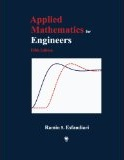## Inside: Applied Mathematics for Engineers, Fifth EditionApplied Mathematics for Engineers, Fifth Edition

## Chapter 1 Complex Numbers, Variables, and Functions

• 1.1 Introduction
• 1.2 Polar form of Complex Numbers
• 1.3 Limit, Continuity, and Differentiability
• 1.4 Analytic Functions, Cauchy-Riemann Equations
• 1.5 Exponential Function
• 1.6 Trigonometric and Hyperbolic Functions
• 1.7 Logarithmic Function
• Problem Set

## Chapter 2 First-Order, Ordinary Differential Equations

• 2.1 Introduction
• 2.2 Mathematical Models of Dynamic Systems
• 2.3 Separable, First-Order ODEs
• 2.4 Exact, First-Order ODEs
• 2.5 Linear, First-Order ODEs and Applications
• 2.6 Orthogonal Trajectories
• Problem Set

## Chapter 3 Second and Higher-Order, Ordinary Differential Equations

• 3.1 Introduction
• 3.2 Homogeneous, Second-Order ODEs with Constant Coefficients and Applications
• 3.3 Second-Order, Euler-Cauchy Differential Equation
• 3.4 Nonhomogeneous, Linear Second-Order ODEs with Constant Coefficients and Applications
• 3.5 Method of Variation of Parameters, Green's Function
• 3.6 Homogeneous, Linear Higher-Order ODEs
• 3.7 Nonhomogeneous, Linear Higher-Order ODEs
• Problem Set

## Chapter 4 Series Solution of Ordinary Differential Equations

• 4.1 Introduction
• 4.2 Series Solution Method
• 4.3 Legendres Equation and Polynomials
• 4.4 Frobenius Method
• 4.5 Bessels Equation and Functions
• 4.6 Differential Equations Satisfied by Bessel Functions
• 4.7 Orthogonal Functions
• Problem Set

## Chapter 5 Laplace Transformation

• 5.1 Introduction
• 5.2 Special Functions
• 5.3 Laplace Transform of Derivatives and Integrals
• 5.4 Inverse Laplace Transformation
• 5.5 Periodic Functions
• 5.6 System Response
• Table 5.1 - Laplace Transform Pairs
• Problem Set

## Chapter 6 Fourier Analysis

• 6.1 Introduction
• 6.2 Steady-State Response via Fourier Series
• 6.3 Fourier Integral
• 6.4 Fourier Transforms
• 6.5 Inverse Fourier Transformation
• Table 6.2 Fourier Transform Pairs
• Tables 6.3, 6.4 Fourier Cosine and Sine Transform Pairs
• Problem Set

## Chapter 7 Partial Differential Equations

• 7.1 Introduction
• 7.2 One-Dimensional Wave Equation, Free Vibration of an Elastic String
• 7.3 Free Vibration of a Uniform Beam
• 7.4 One-Dimensional Heat Equation, Two-Dimensional Steady-State Heat Flow
• 7.5 Two-Dimensional Wave Equation, Vibration of Membranes
• 7.6 Steady-State Heat Conduction in a Solid Sphere
• 7.7 Solution of PDEs via Laplace Transformation
• Problem Set

## Chapter 8 Matrix Analysis

• 8.1 Introduction
• 8.2 Linear Systems of Equations
• 8.3 Determinant of a Matrix
• 8.4 Inverse of a Matrix
• Problem Set

## Chapter 9 Matrix Eigenvalue Problem

• 9.1 Introduction
• 9.2 Eigenvalues of Special Matrices
• 9.3 Matrix Diagonalization
• 9.4 Modal Decomposition
• 9.5 Simultaneous Diagonalization
• 9.6 Systems of Linear, First-Order Differential Equations
• 9.7 State Variables
• Problem Set

## Chapter 10 Introduction to MATLAB®

• 10.1 Built-in Functions
• 10.2 Vectors and Matrices
• 10.3 User-Defined Functions and Script Files
• 10.4 Flow Control
• 10.5 Data Formatting
• 10.6 Symbolic Toolbox
• 10.7 Plotting
• Problem Set

## Chapter 11 Numerical Solution of Equations and Systems

• 11.1 Introduction to Numerical Methods
• Solution of Equations of a Single Variable
• 11.2 Bracketing Methods
• 11.3 Fixed-Point Method
• 11.4 Newton's Method (Newton-Raphson Method)
• 11.5 Secant Method
• Solution of Linear Systems of Equations
• 11.6 Gauss Elimination Method
• 11.7 LU Factorization Methods
• 11.8 Iterative Solution of Linear Systems
• 11.9 Ill-Conditioning and Error Analysis
• 11.10 Systems of Nonlinear Equations
• Problem Set

## Chapter 12 Curve Fitting and Interpolation

• 12.1 Linear Regression
• 12.2 Linearization of Nonlinear Data
• 12.3 Polynomial Regression
• 12.4 Polynomial Interpolation
• 12.5 Spline Interpolation
• 12.6 Fourier Approximation and Interpolation
• Problem Set

## Chapter 13 Numerical Differentiation and Integration

• 13.1 Finite-Difference Formulas for Numerical Differentiation
• 13.2 Numerical Integration: Newton-Cotes Formulas
• 13.3 Numerical Integration of Analytical Functions: Gaussian Quadrature
• Problem Set

## Chapter 14 Numerical Solution of Initial- and Boundary-Value Problems

• Initial-Value Problems
• 14.1 Euler's Method
• 14.2 Runge-Kutta Methods
• 14.3 Multistep Methods
• 14.4 Systems of Ordinary Differential Equations
• 14.5 MATLAB Built-in Functions for Initial-Value Problems
• Boundary-Value Problems
• 14.6 Shooting Method
• 14.7 Finite-Difference Method
• 14.8 MATLAB Built-in Function bvp4c for Boundary-Value Problems
• Problem Set

## Chapter 15 Numerical Methods for Eigenvalue Approximation

• 15.1 Power Method - Estimation of the Dominant Eigenvalue
• 15.2 Deflation Methods
• 15.3 Householder Tridiagonalization and QR Factorization Methods
• Problem Set

## Chapter 16 Numerical Solution of Partial Differential Equations

• 16.1 Elliptic Partial Differential Equations
• 16.2 Parabolic Partial Differential Equations
• 16.3 Hyperbolic Partial Differential Equations
• Problem Set

## Appendix 3 Tables

• Table 1 Gamma Function
• Table 2A Bessel Functions of the First Kind
• Table 2B Bessel Functions of the Second Kind